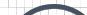Informative Information for the Uninformed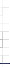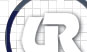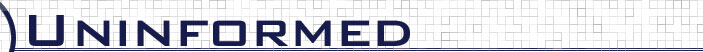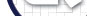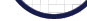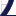Vol 5» 2006.Sep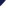# Modified BayesCompare Metric

Another variant of BayesCompare was investigated. As pointed out above, conditional probability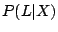can become zero if L has a duration that has not yet been learned to be producible by implementation X, perhaps because the print database hasn't been updated for some time. So another version was explored where only duration values that fall in the intersection of an input fingerprint L and a database fingerprint R are included in the calculation of. So the product becomes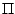R.duration_ratio(d) where d ranges over all durations in L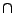R, andR.duration_ratio(p, d) where p and d range over all packet types and durations respectively where duration d occurs in a packet of type p in LR.

Figure 4.9: BayesCompare-Modified duration value only analysis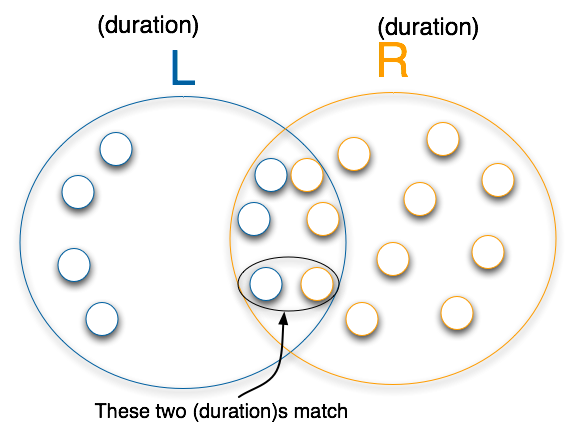ret = 1.0
for every duration-value d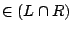ret *= R.duration_ratio(d)
return ret;



Figure 4.10: BayesCompare-Modified (packet-type, duration) analysis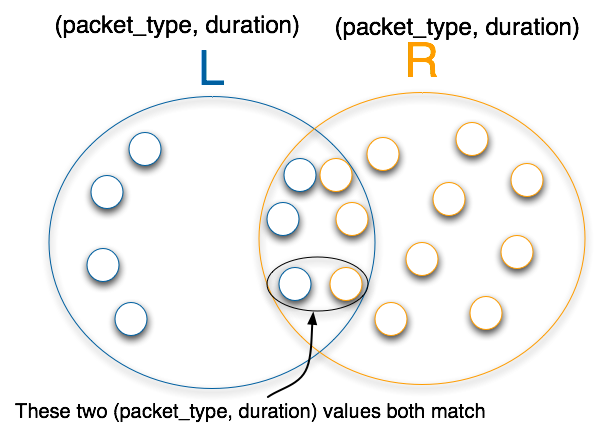ret = 1.0
for every packet_type p, duration-value dret *= R.duration_ratio(p, d)
return ret;